Last week we collected some lexical decision data and started manipulating and plotting it so we’re going to jump right back in where we left off. Start by loading up the libraries and data in the same way as we did last week.

library(tidyverse)
data_source <- "http://web.mit.edu/psycholinglab/data/"
rt_data <- read_csv(file.path(data_source, "in_lab_rts_2018.csv"))

## Data visualization, continued

What hypothesis do we want to test about the data? Fake words take longer than Real words to identify.

So we’re going to try to address this by visualizing our data. The first step is to add the relevant information to our dataframe. For that we’re going to refer back to some of the tidyr/dplyr functions.

Try it yourself: Add a column, “word_type”, to d that says if a word is real or fake

words <- unique(rt_data$word) real_words <- words[c(1, 3, 5, 7, 8, 10, 12, 15, 17, 18, 21, 22)] real_words ##  "book" "eat" "condition" "word" ##  "retire" "fly" "coat" "art" ##  "gold" "three" "encyclopedia" "understandable" rt_data <- rt_data %>% mutate(word_type = if_else(word %in% real_words, "Real", "Fake")) Previously we saw that we could look at distributions using geom_histogram ggplot(rt_data) + geom_histogram(aes(x = rt), bins = 60, color = "white")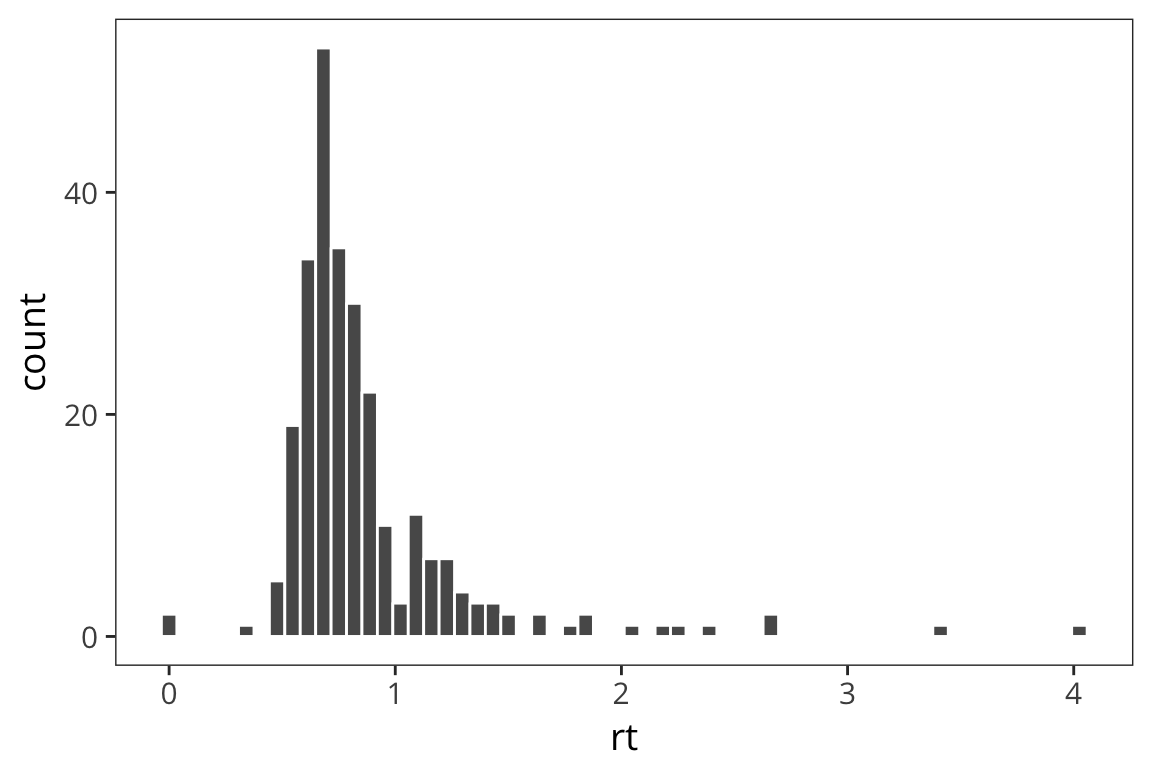Another way to look at the distribution is to plot the density with geom_density() ggplot(rt_data) + geom_density(aes(x = rt), fill = "blue", alpha = 0.2)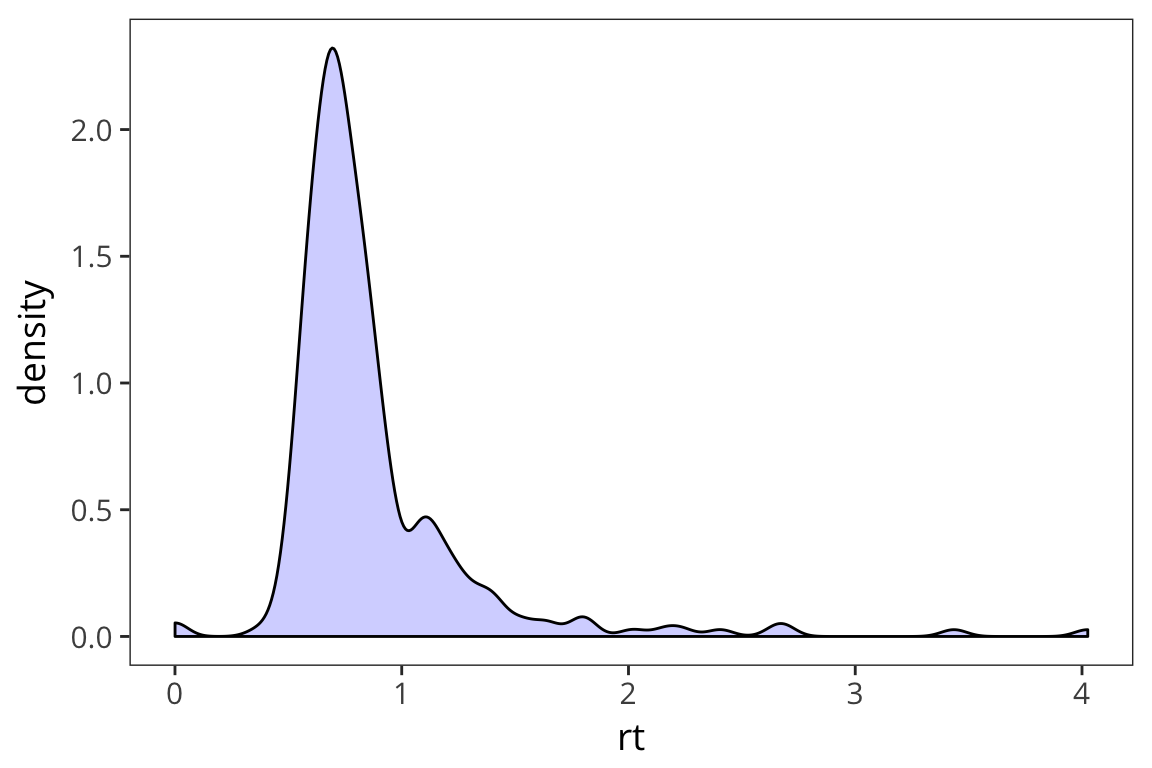### Facets Now we want to start addressing our question about how RTs may differ for real vs. fake words. One way to do this is to split the RT data we have into Real and Fake and plot the data side by side. ggplot has an easy way to do that with facetting. ggplot(rt_data) + geom_histogram(aes(x = rt), bins = 60, color = "white") + facet_wrap(~word_type)facet_wrap() includes a ~ followed by the variable you want to split your data by. Faceting works with any geom and is a great way to de-clutter a plot. ggplot(rt_data) + geom_density(aes(x = rt), fill = "blue", alpha = 0.2) + facet_wrap(~word_type)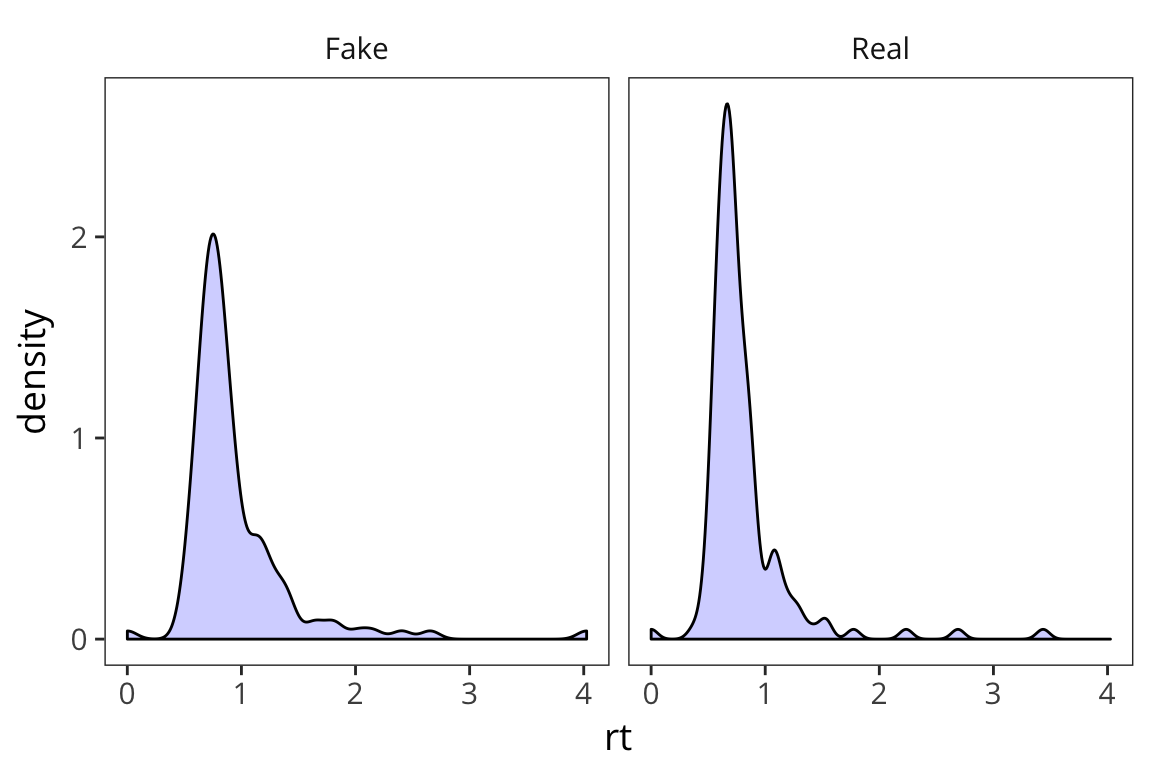What do you observe? This is informative but it’s not the easiest way to really draw a comparison in this case. ### Boxplots We want to aggregate the data but maintain some information about its variability. We can use a boxplot which plots the quartiles of the distribution (2nd quartile = median). ggplot(rt_data) + geom_boxplot(aes(x = word_type, y = rt))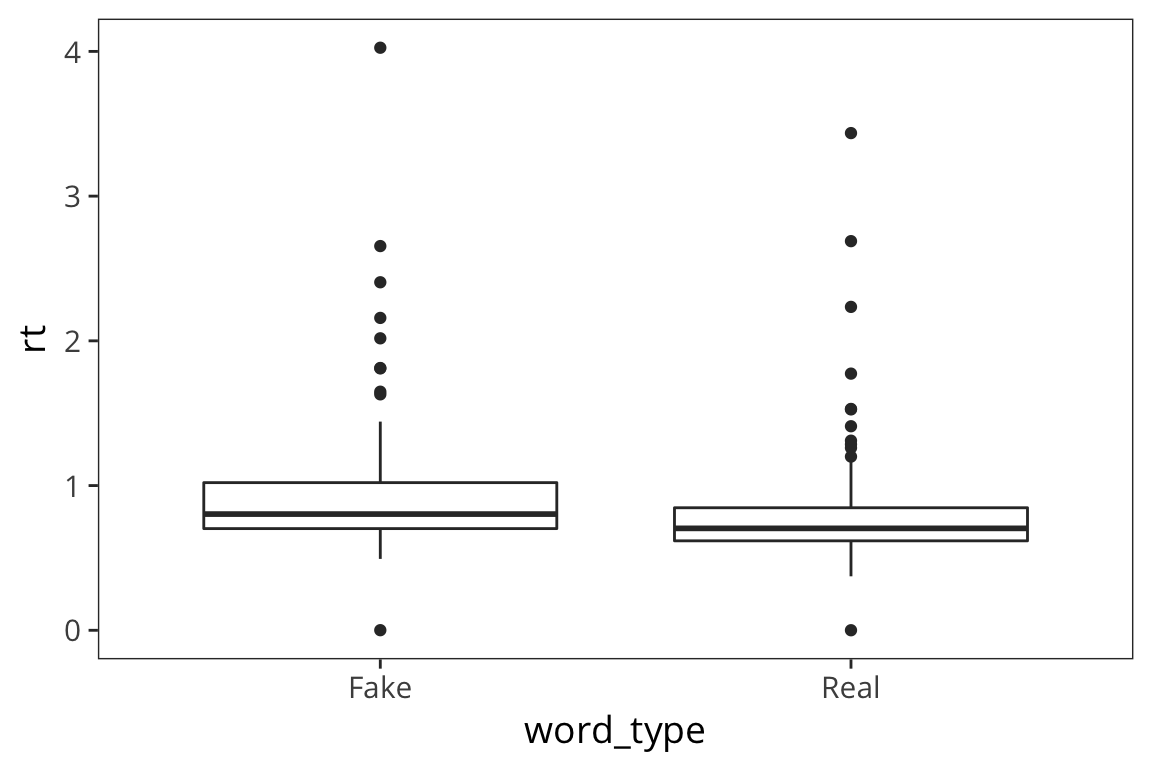### Violin plots Another, more aesthetically pleasing way to plot this is with violin plots ggplot(rt_data) + geom_violin(aes(x = word_type, y = rt, fill = word_type))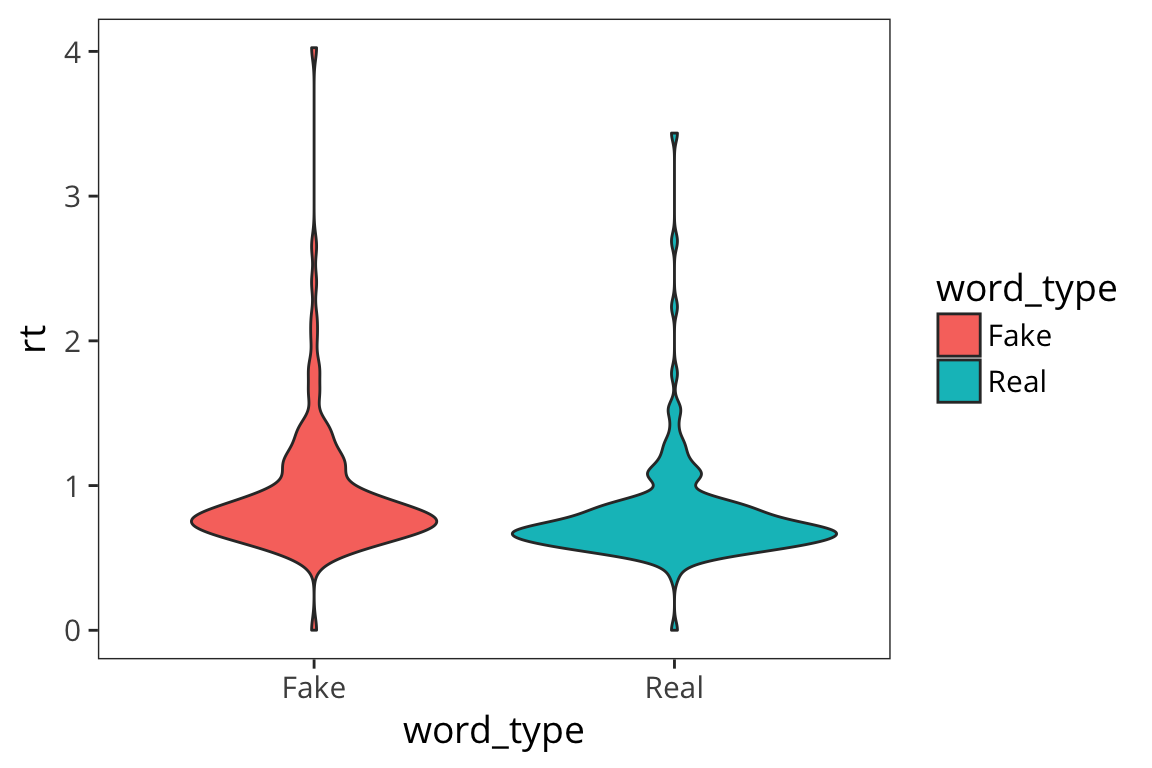What can we learn from this boxplot? It looks like real words have faster median RTs and somewhat less variability (except for that 1 outlier). ### Barplots Another commonly way to plot this is with a barplot. Bar plots are very popular possibly because they allow you to plot the mean which is a statistic that people find really useful, but they actually obscure a lot of information about the distribution. I“m still showing you how to make them because they are so widespread. rt_by_real <- rt_data %>% group_by(word_type) %>% summarise(mean_rt = mean(rt)) ggplot(rt_by_real) + geom_col(aes(x = word_type, y = mean_rt, fill = word_type))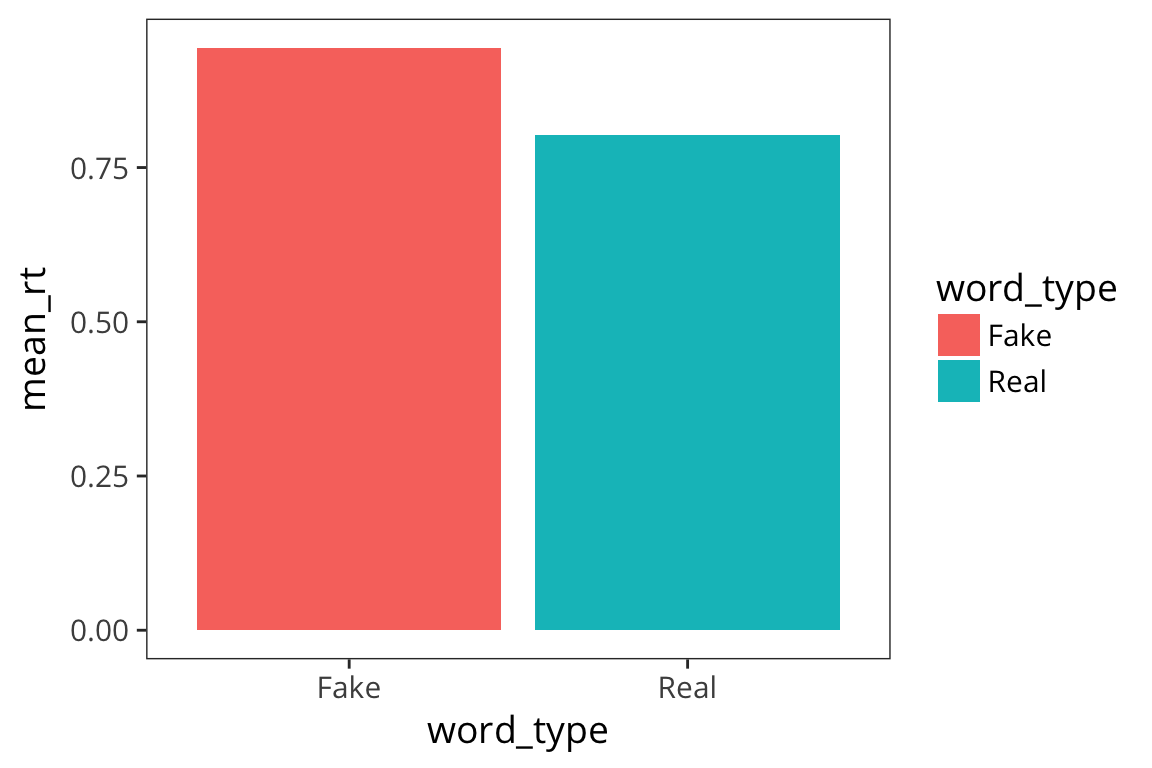This again shows us that fake words lead to slower responses but we have no information about how variable the data are within each bar. This is one of the main problems with using bar charts. To try to get at the variability, people often use error bars (which I will show you how to add after we discuss standard error and confidence intervals). ### Scatter plots Now what other variables can we think of which would affect RTs? Let’s look at mean RTs by word. ### Try it yourself… 1. Collapse the data to get the mean RT for each word. 2. Add a column “word_length” that has the length of the word. rt_words <- rt_data %>% group_by(word, word_type) %>% summarise(word_rt = mean(rt)) %>% mutate(word_length = str_length(word)) Let’s plot word RT against word length. ggplot(rt_words, aes(x = reorder(word, word_rt), y = word_rt)) + geom_point()The axis is impossible to read and map onto the dots, so let’s change it. ggplot(rt_words, aes(x = reorder(word, word_rt), y = word_rt)) + geom_point() + theme(axis.text.x = element_text(angle = 45, hjust = 1))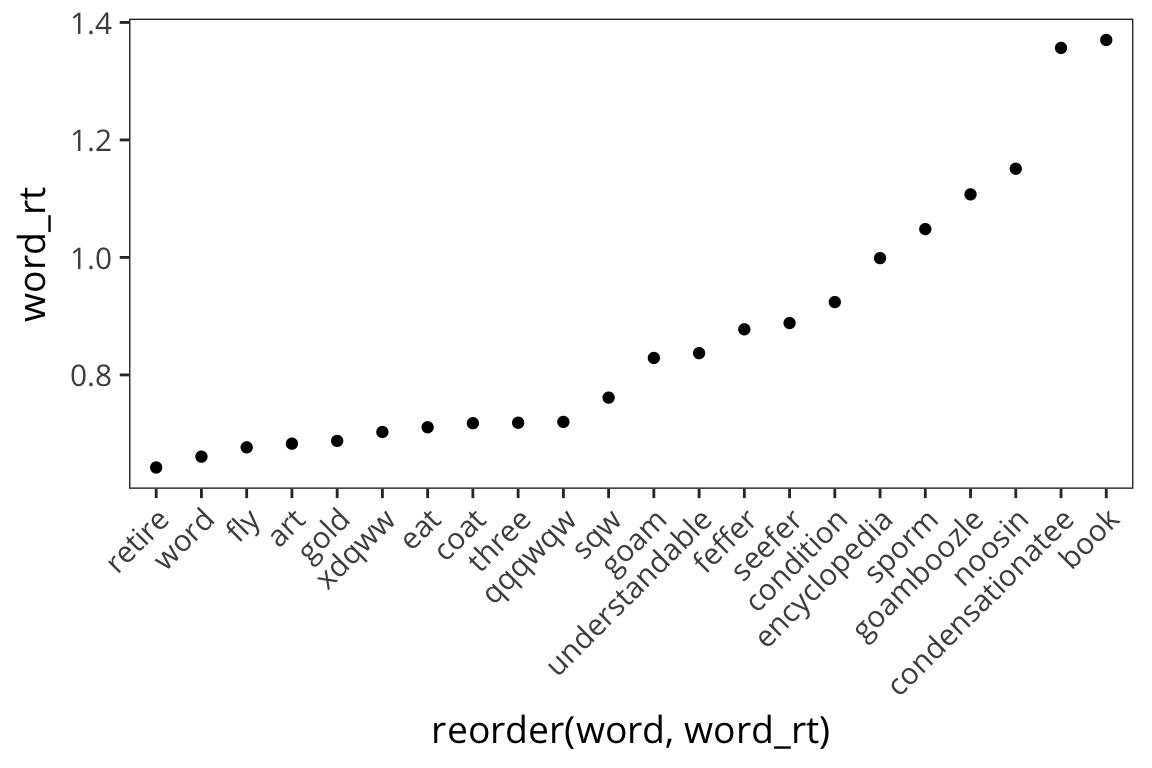Okay, what do we see now? Looks like long words have longer RTs. ggplot(rt_words, aes(x = word_length, y = word_rt)) + geom_text(aes(label = word))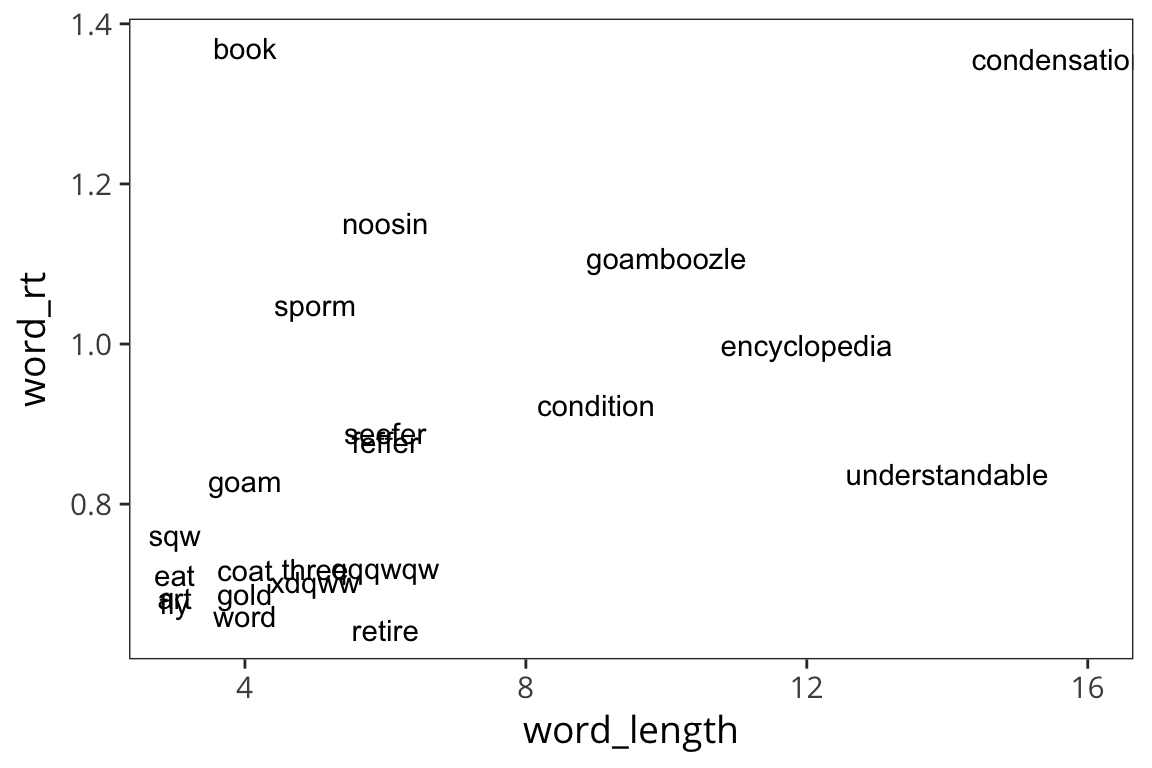ggplot(rt_words, aes(x = word_length, y = word_rt)) + geom_text(aes(label = word)) + geom_smooth(method = lm)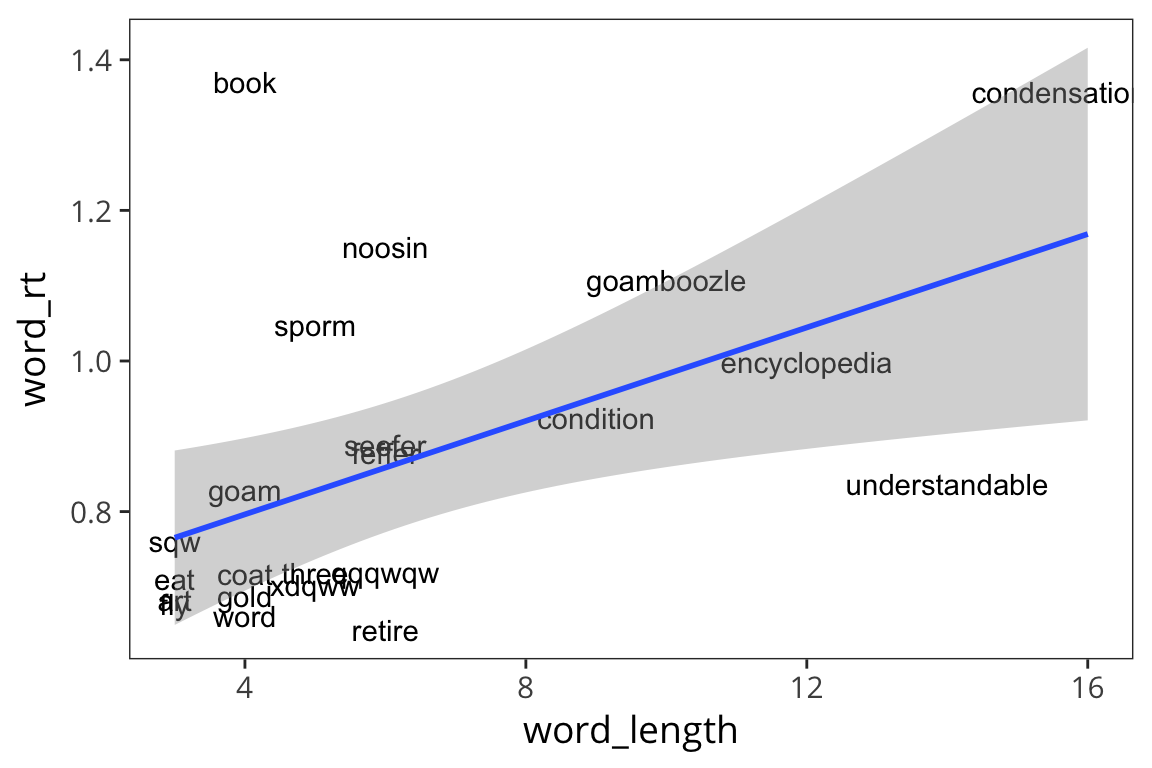So it does look like there is a relationship there, such that long words take longer to respond to. But we’re mixing together real and fake words and we saw previously that those lead to different average RTs so let’s see if length has a different effect on real words vs. fake words. ggplot(rt_words, aes(x = word_length, y = word_rt, label = word)) + geom_text() + geom_smooth(method = lm) + facet_wrap(~word_type)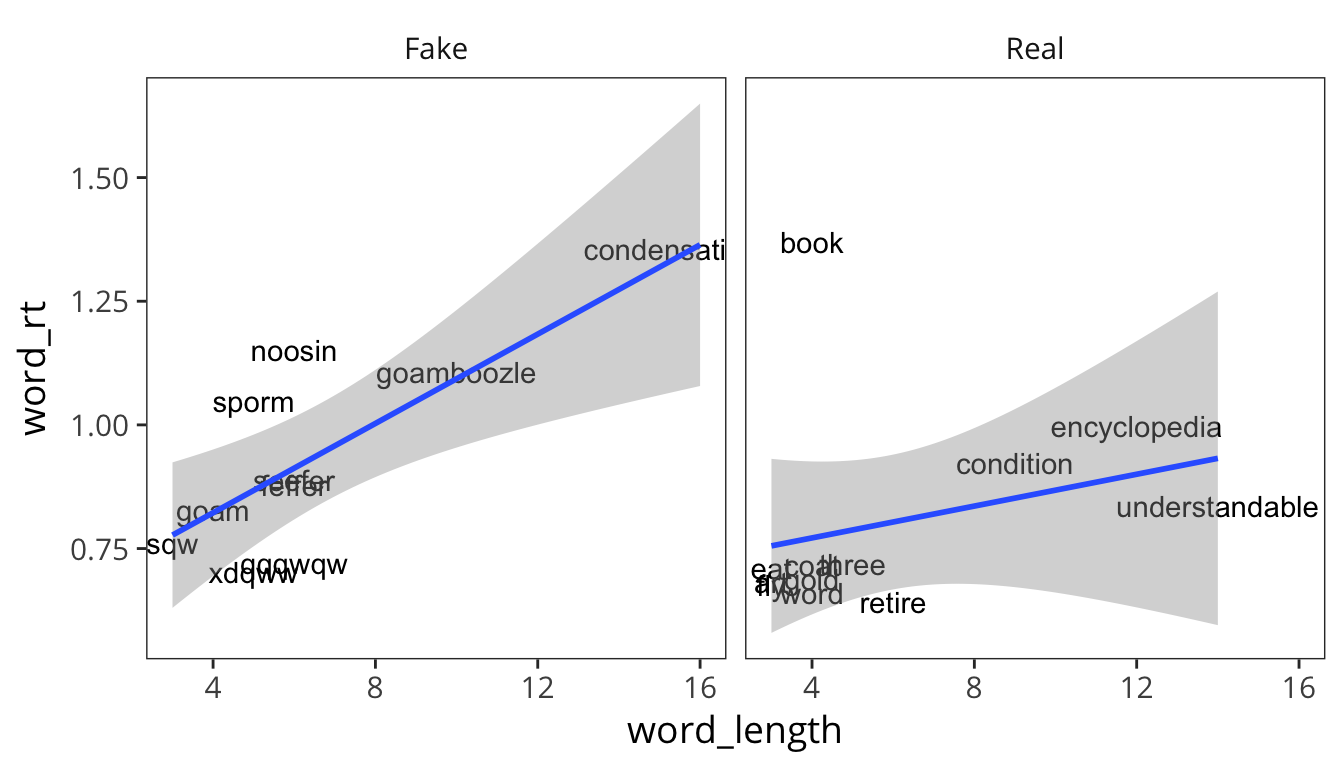Looks like the relationship between length and RT is bigger for Fake words than Real words. We might also want to look at how speed of response is associated with how closely a word resembles a word in English. So let’s categorize the fake words based on some metric of similarity to real words. rt_fake <- rt_words %>% filter(word_type == "Fake") rt_fake$word
##   "condensationatee" "feffer"           "goam"
##   "goamboozle"       "noosin"           "qqqwqw"
##   "seefer"           "sporm"            "sqw"
##  "xdqww"
similar <- rt_fake$word[c(1, 2, 3, 4, 5, 7, 8)] rt_fake <- rt_fake %>% mutate(similar_to_real = word %in% similar) ggplot(rt_fake, aes(x = word_length, y = word_rt)) + geom_text(aes(label = word)) + geom_smooth(method = lm) + facet_wrap(~similar_to_real)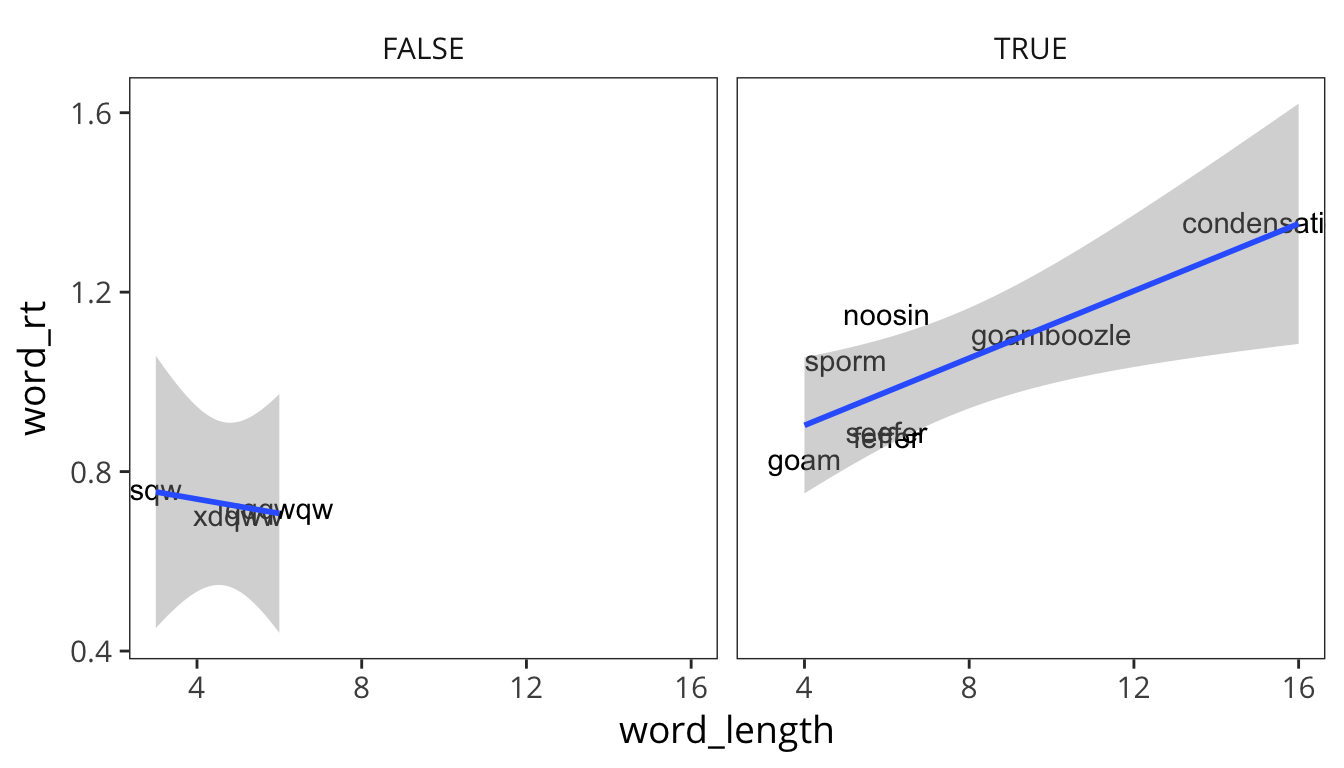So it looks like maybe there is an effect of length in the similar-to-real words but not the very fake words. Of course, this is all completely post-hoc and exploratory. There’s clearly not enough data here to make any claims and the materials haven’t been carefully controlled or balanced. This is just to illustrate how you can go back and forth between hypothesizing and data visualization to get a good sense of patterns in your data, before you do any statistical analysis. ### Try it yourself… 1. Read in the following file: http://web.mit.edu/psycholinglab/www/data/four_datasets.csv It contains 4 datasets (A,B,C,D) and x and y values for each. 2. For each dataset, get: • the mean of x • the mean of y • the standard deviation of x • the standard deviation of y • the correlation of x and y (using cor()) 3. Using facet_wrap() make a figure with 4 scatterplots, one for each dataset. What do you observe? datasets <- read_csv(file.path(data_source, "four_datasets.csv")) datasets_summary <- datasets %>% group_by(dataset) %>% summarise(mean_x = mean(x), mean_y = mean(y), sd_x = sd(x), sd_y = sd(y), cor_xy = cor(x, y)) datasets_summary ## # A tibble: 4 x 6 ## dataset mean_x mean_y sd_x sd_y cor_xy ## <chr> <dbl> <dbl> <dbl> <dbl> <dbl> ## 1 A 9 7.500909 3.316625 2.031568 0.8164205 ## 2 B 9 7.500909 3.316625 2.031657 0.8162365 ## 3 C 9 7.500000 3.316625 2.030424 0.8162867 ## 4 D 9 7.500909 3.316625 2.030579 0.8165214 ggplot(datasets) + geom_point(aes(x = x, y = y)) + facet_wrap(~dataset)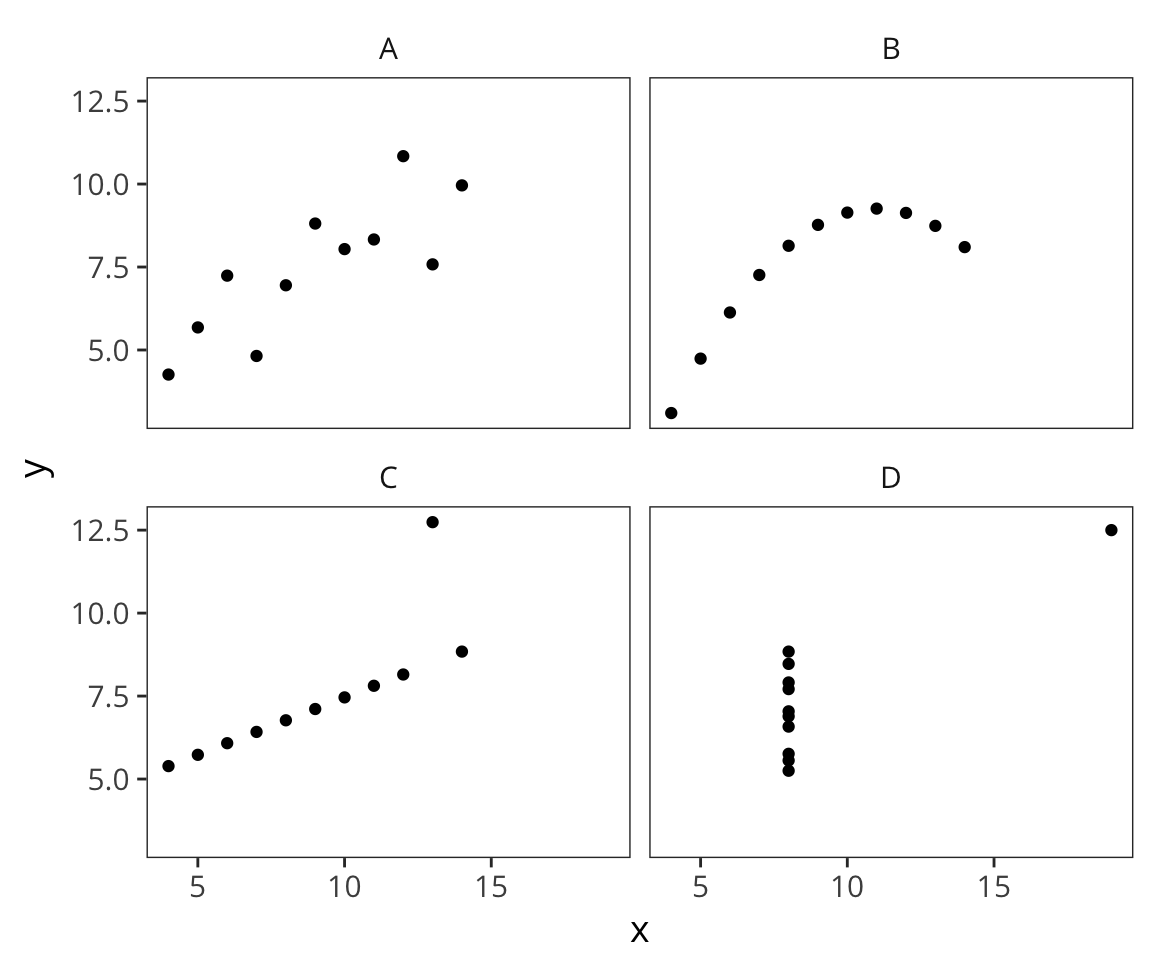ggplot(datasets, aes(x = x, y = y)) + geom_point() + geom_smooth(method = lm) + facet_wrap(~dataset)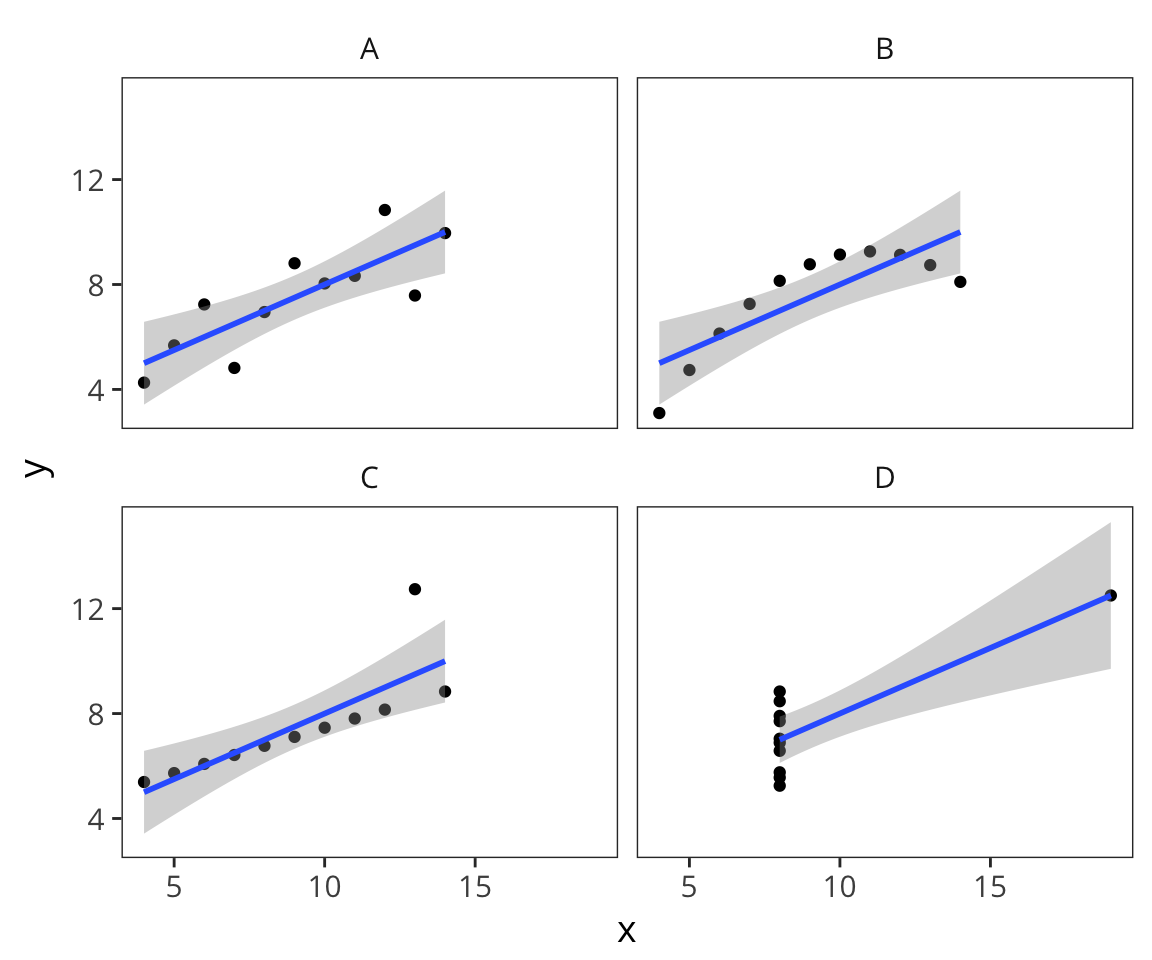See Anscombe’s quartet on Wikipedia for more details about these data. There’s also a huge number of ways to customize the appearance of your graphs which I won’t get into now but you can go to Help –> Cheatsheets –> Data visualization with ggplot2. ## Statistics ### Summary statistics So we’ve been using mean() and sd() already but let’s think some more about what they actually represent and why they’re important. ggplot(rt_data) + geom_density(aes(x = rt), fill = "blue", alpha = 0.2) + facet_wrap(~subject)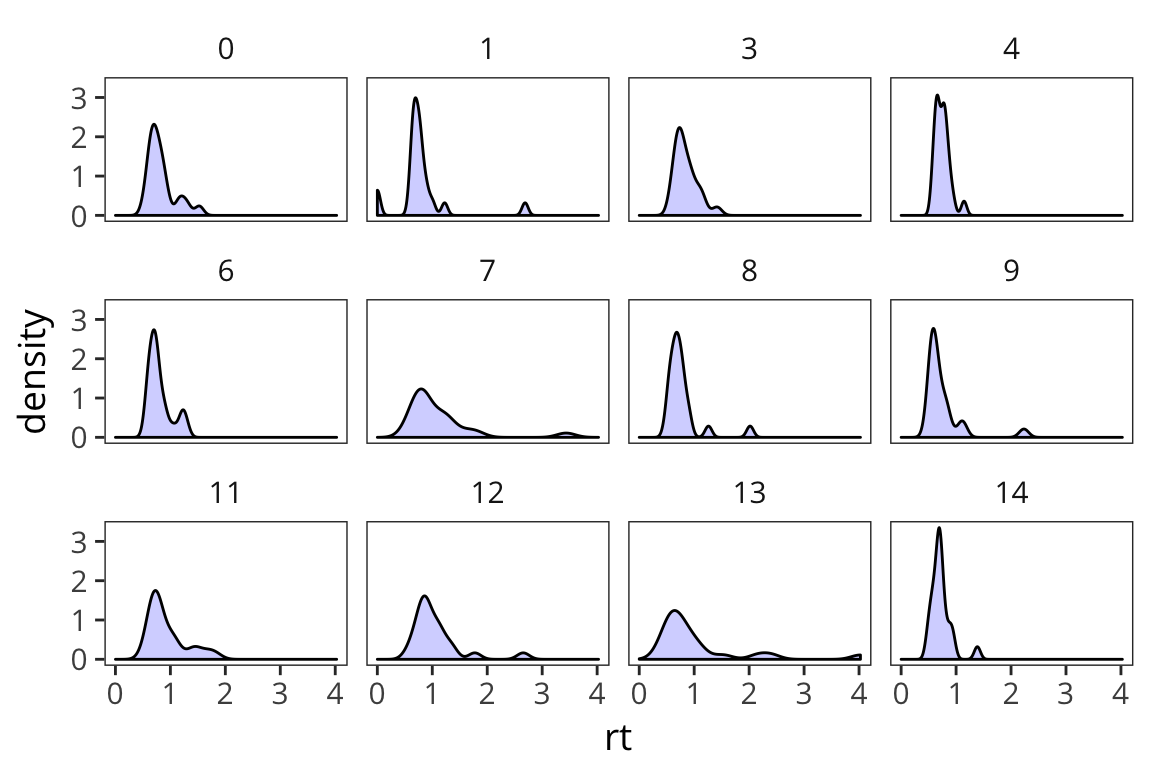You can see that even in this tiny sample there is quite a bit of variability in terms of how RTs are distributed. And you can sort of see that if you were to try and characterize these distributions, 2 big pieces of information would be central tendency and spread (there’s also things like skew and kurtosis but they’re much less used). Arithmetic Mean and Median tell us about the central tendency of the data. What about the spread of the data? First thing that might come to mind? How about the mean distance from the mean? Let’s try this out by generating a bunch of random numbers now in R from a normal distribution using rnorm(). random_numbers <- data_frame(nums = rnorm(10000)) ggplot(random_numbers) + geom_histogram(aes(x = nums), bins = 60, color = "white")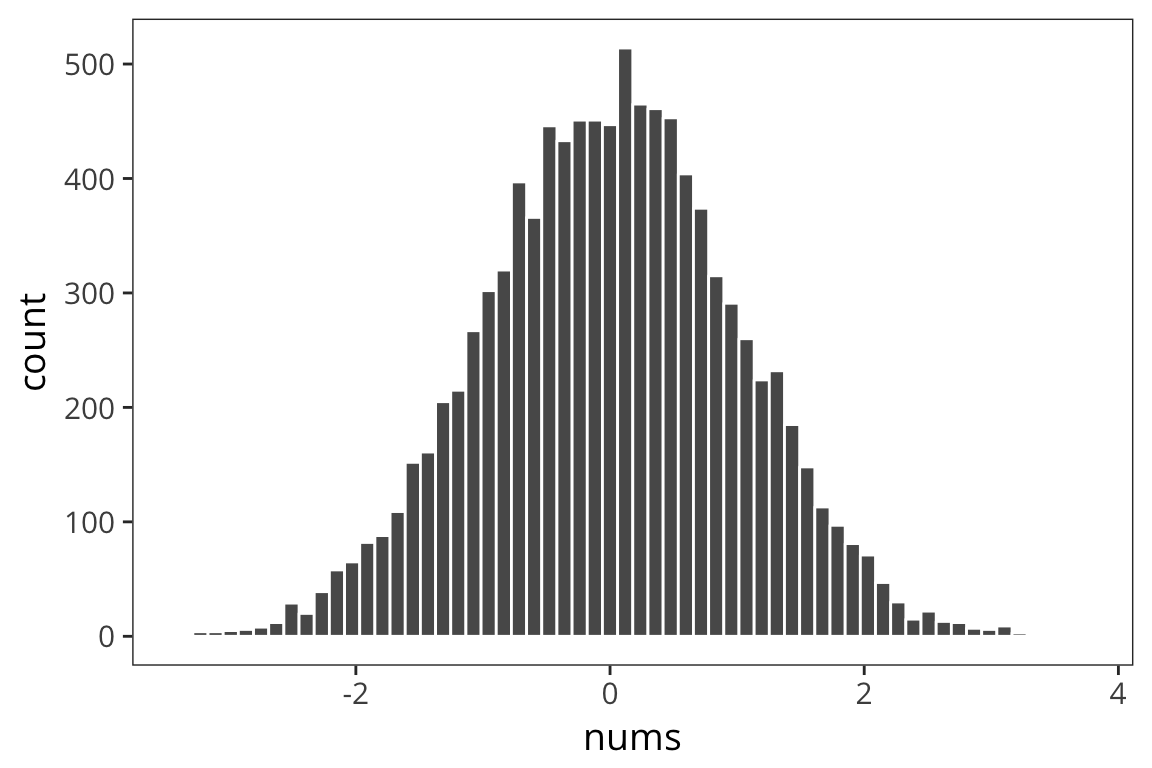We see our typical Gaussian/bell-curve: most numbers are near zero, and they thin out as you get farther away from zero. We see the mean is zero, or pretty close: mean(random_numbers$nums)
##  0.009460357

Let’s look at the mean of mean absolute differences from zero:

mean(abs(random_numbers$nums - mean(random_numbers$nums)))
##  0.7972852

The benefit of the absolute value is to throw away the fact that some things are to the left and others are to the right of the mean. This is called the absolute variance.

But it turns out a similar measure has much nicer mathematical properties down the line. Let’s instead look at the average SQUARED distance to the mean.

sum((random_numbers$nums - mean(random_numbers$nums)) ^ 2) /
nrow(random_numbers)
##  0.9961713

This is called VARIANCE. The square root of variance is called STANDARD DEVIATION or sd. It has a lot of nice properties. You can see one of them here: for Normal-distributed data, the SD is 1.

sqrt(sum((random_numbers$nums - mean(random_numbers$nums)) ^ 2) /
nrow(random_numbers))
##  0.9980838

We can get the variance and standard deviation using var() and sd()

var(random_numbers$nums) ##  0.9962709 sd(random_numbers$nums)
##  0.9981337

Note that the number you get out of this is SLIGHTLY difference than the one you got out of the formula. We’ll go into why that is later today. Here is the formula which you should use, which gives you the same numbers

sum((random_numbers$nums - mean(random_numbers$nums)) ^ 2) /
(length(random_numbers$nums) - 1) ##  0.9962709 sqrt(sum((random_numbers$nums - mean(random_numbers$nums)) ^ 2) / (length(random_numbers$nums) - 1))
##  0.9981337

Now we have measures of central tendency and spread. Remember that we have data that look like this.

ggplot(rt_data) +
geom_density(aes(x = rt), fill = "blue", alpha = 0.2) +
facet_wrap(~subject)For a high-variance subject, something that’s x far from the mean might be equivalent to something that’s y far from the mean for a low-variance subject. We might have similar effects here, just on different scales. We can use mean and standard deviation to roughly put all the data on the same scale. This is called z-scoring.

### Writing functions

Before I show you how to compute z-scores, we’ll make a slight detour to learn about writing functions in R.

You’ve seen me use a lot of functions that are either part of base R or part of Hadley Wickham’s tidyverse. But let’s say you want to do something for which no one has yet written a function. What can you do? Make your own function!

Let’s make a silly function, which takes an argument x, and returns x + 1.

silly_function <- function(x) {
return(x + 1)
}

silly_function(7)
##  8

You can put any series of expressions you want in a function.

silly_function_2 <- function(x) {
a <- c(1, 2, 3)
return(a + x)
}

silly_function_2(7)
##   8  9 10

Functions can take any type of arguments and return any type of values.

Within the functions (or independently) you can use control structures like an IF STATEMENT. This lets you do something IF some expression is TRUE. (similar to if_else())

silly_function_3 <- function(x) {
if (x > 50) {
return("x is greater than 50")
}
}

silly_function_3(100)
##  "x is greater than 50"
silly_function_3(20)

We can add an additional clause to this statement, which is something to do OTHERWISE:

silly_function_4 <- function(x) {
if (x > 50) {
return("x is greater than 50")
} else if (x < 50) {
return("x is less than 50")
}
}

silly_function_4(100)
##  "x is greater than 50"
silly_function_4(20)
##  "x is less than 50"

We can chain together lots of if statements…

silly_function_5 <- function(x){
if (x > 50) {
return("x is greater than 50")
} else if (x < 50) {
return("x is less than 50")
} else {
return("x is 50")
}
}

silly_function_5(100)
##  "x is greater than 50"
silly_function_5(20)
##  "x is less than 50"
silly_function_5(50)
##  "x is 50"

### Try it yourself…

1. Write a function that takes a vector of numbers as an argument and returns that vector’s mean and standard deviation.

2. Use your function to get the mean and sd of random_numbers$nums. summary_stats <- function(x) { mean_x <- mean(x) sd_x <- sd(x) stats <- c("mean" = mean_x, "sd" = sd_x) return(stats) } summary_stats(random_numbers$nums)
##        mean          sd
## 0.009460357 0.998133722

### Z-scoring

z_score <- function(x) {
return( (x - mean(x)) / sd(x) )
}

This says first center your values around the mean value, then divide by SD to stretch out or shrink your space in standardized way. You’re normalizing your scale here so the units are standard deviations: if your z-score is 1, that means that the original datapoint was one standard deviation away from the mean. The idea is that distances in terms of SD are comparable across groups which differ in mean and variance in a way that raw values are not.

rt_data <- rt_data %>% mutate(z_rt = z_score(rt))

ggplot(rt_data) +
geom_histogram(aes(x = rt), bins = 60, color = "white")ggplot(rt_data) +
geom_histogram(aes(x = z_rt), bins = 60, color = "white")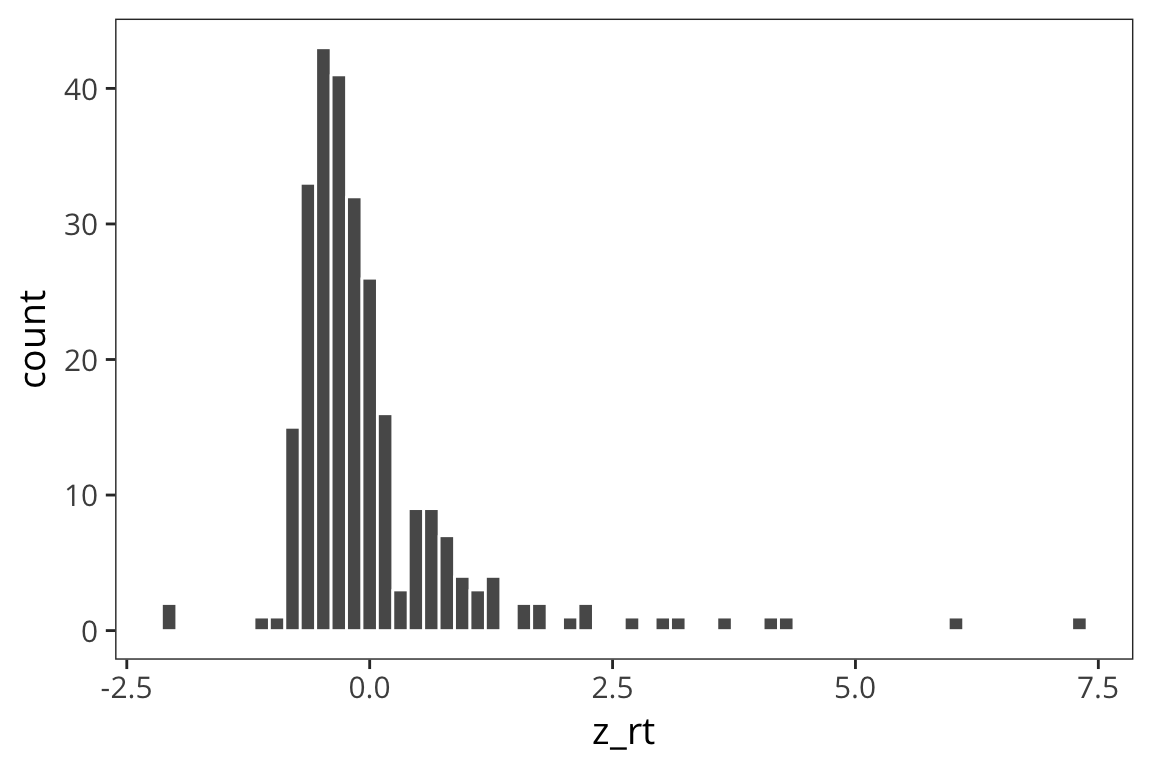You can see that the z scores are centered on 0 and the shape is a little more gaussian but this doesn’t get rid of outliers.

In general, in the context of the normal distribution we expect about 68% of the datapoints to be within 1 standard deviation of the mean.

So if sd(x) = 10, that means that we expect 68% of the datapoints to be between mean - 10 and mean + 10. We expect 96% of the data to be within 2 standard deviations of the mean, and 99% of the data to be within 3 standard deviations of the mean. These numbers may seem strange and random to you right now but it’ll make more sense as we learn more about the Normal.

### The normal distribution

The normal distribution is parameterized by the mean and the standard deviation. When we talk about the parameters of the distribution we’ll call these $$\mu$$ and $$\sigma$$, rather than mean and sd. These are the parameters you would use to generate a specific distribution (e.g., with rnorm).

# generate 1000 random numbers from a normal distribution with mu 0 and sigma 1
rand_nums <- rnorm(1000, 0, 1)
hist(rand_nums)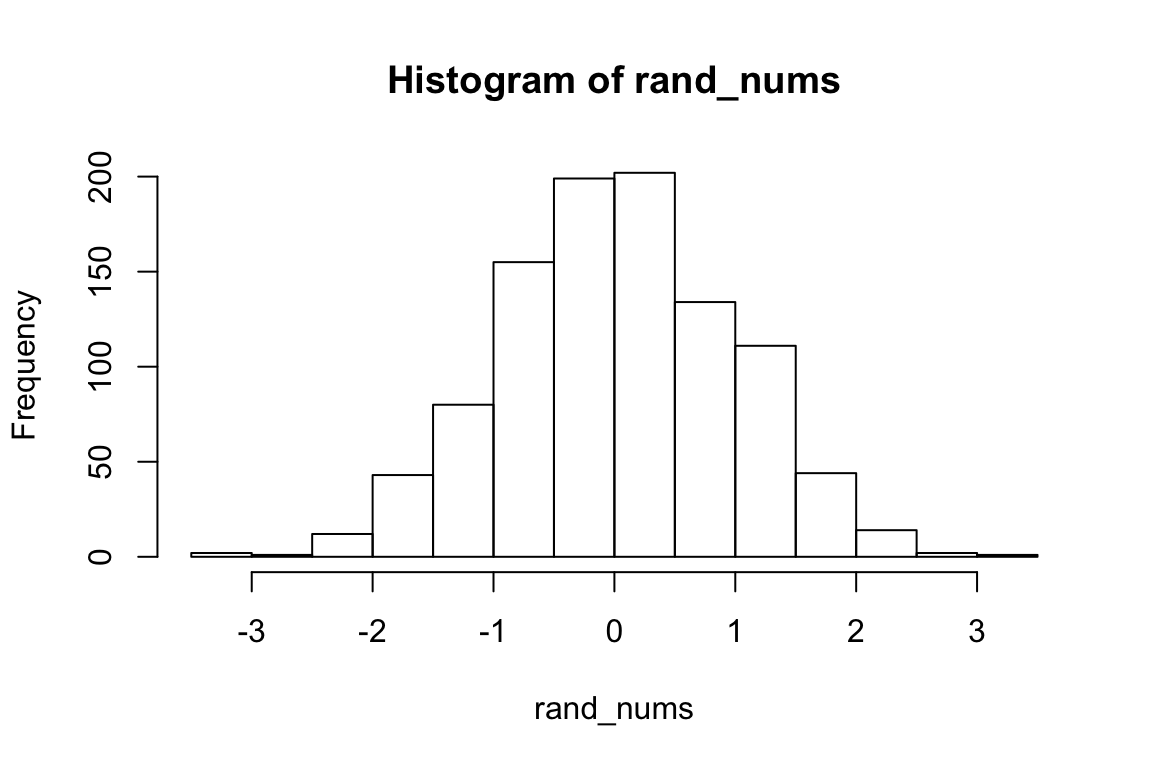We’ll use mean and sd to refer to the actual values computed from the data that are the result of such a generative process.

mean(rand_nums)
##  0.03460842
sd(rand_nums)
##  0.9579582

We see the mean is close to $$\mu$$, and the sd is close to $$\sigma$$ but they are not identical. The mean function provides an ESTIMATE of $$\mu$$, and the sd function provides an ESTIMATE of $$\sigma$$. (This is why we have to use the corrected sd formula; otherwise it would not be a good estimate of $$\sigma$$).

Let’s look at what happens when we change the parameters of the Normal.

hist(rnorm(1000, 50, 1))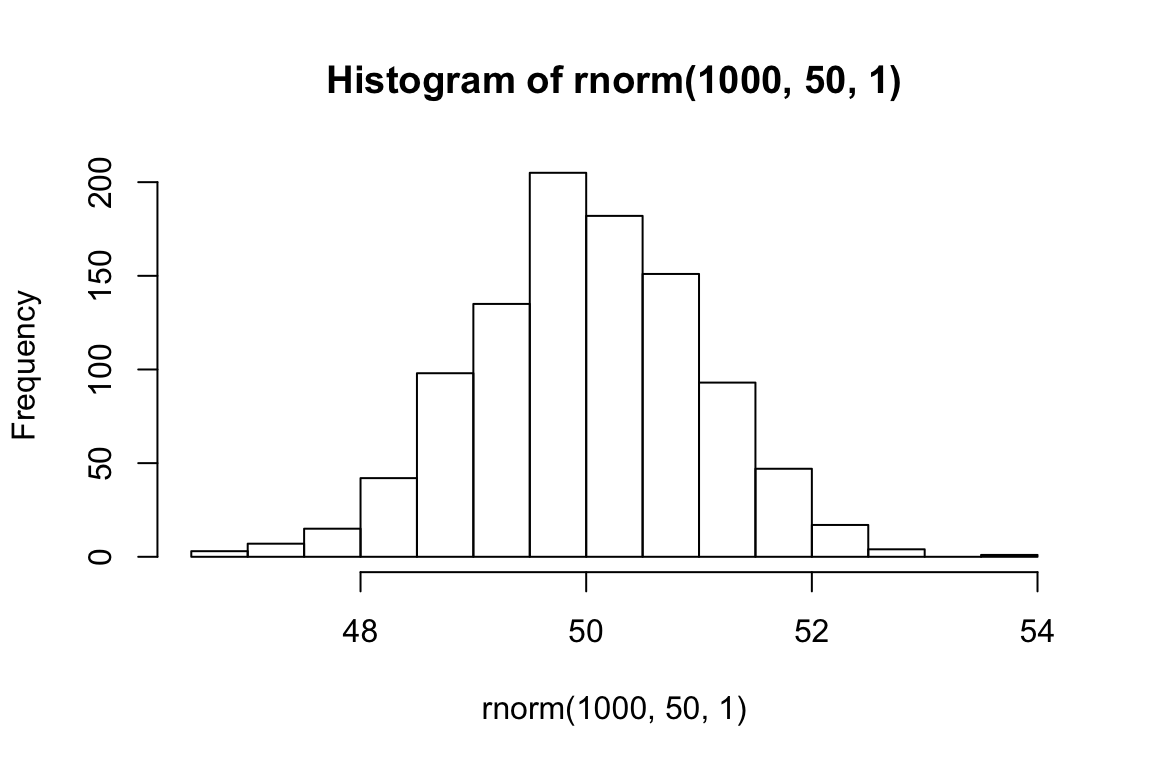mean(rnorm(1000, 50, 1))
##  50.02274
hist(rnorm(1000, 0, 100))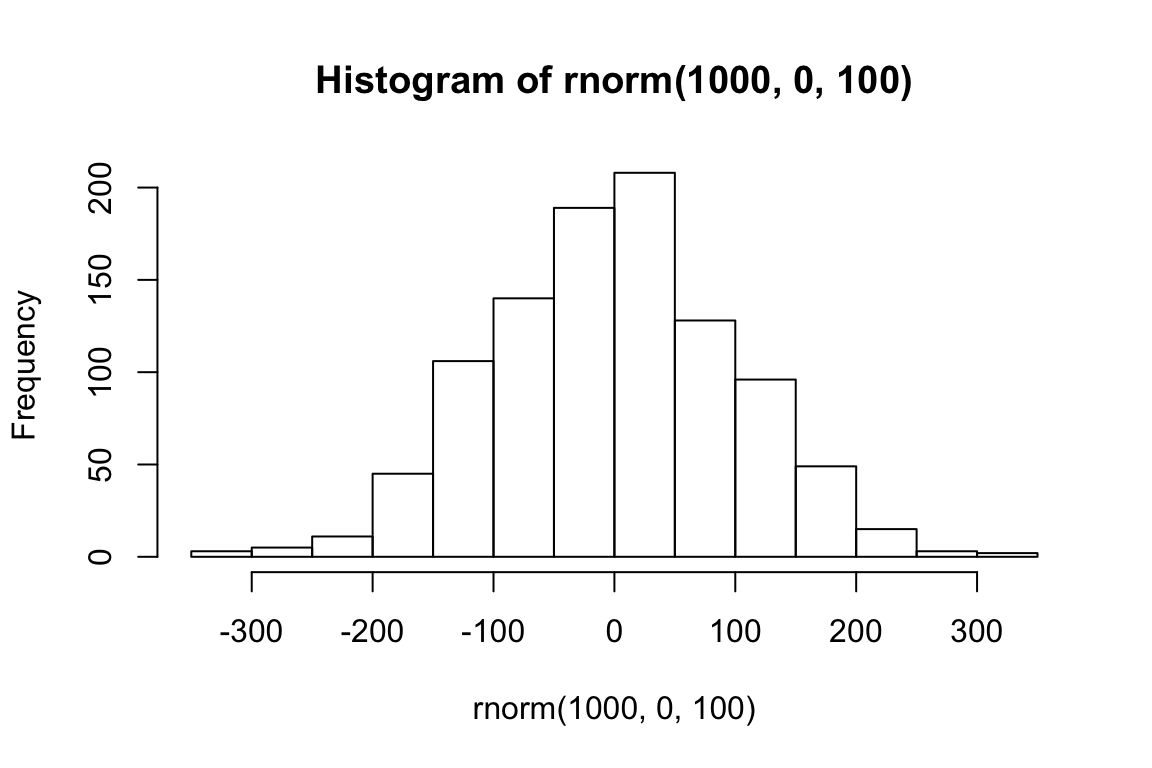sd(rnorm(1000, 0, 100))
##  98.62611

The normal distribution represents a generative process for data. Here it is an R function with two parameters. Say we have lots of datapoints that we know were generated using this function. Now we want to know, what were the values of the two parameters, $$\mu$$ and $$\sigma$$, which were passed into the function in order to generate this data? We can estimate $$\mu$$ using mean(), and $$\sigma$$ using sd().

In general, this is a powerful way to think about statistics, and about science in general: we know there is some function that generated the data in front of us, and we want to guess what were the parameters passed into that function? Or what exactly was the function? Recovering these things is the goal of all inference. In the case of data which is normally-distributed, all you need to recover is these two numbers, and you will have a function which can simulate your data.

Let’s see how this works with real data.

rts <- read_csv(file.path(data_source, "rts.csv"))
hist(rts$RTlexdec)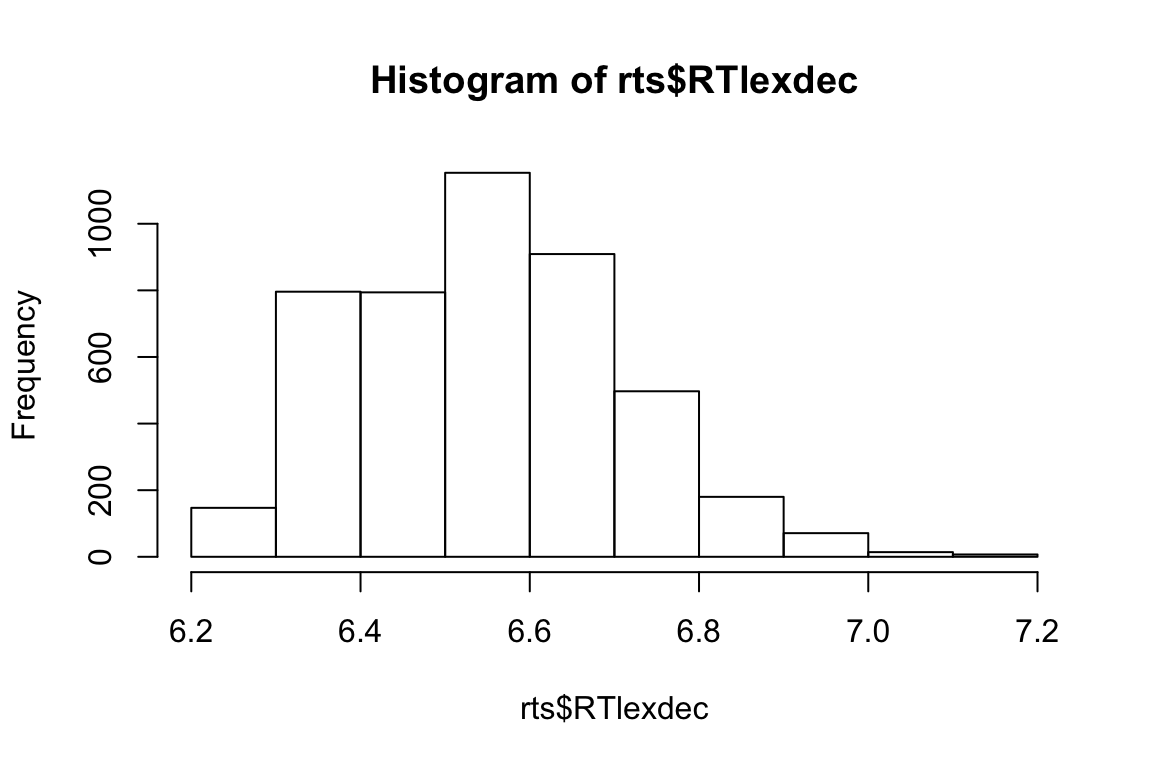This is somewhat normally distributed. Let’s pretend the data were generated by rnorm, and try to figure out what were the arguments to that function. mean(rts$RTlexdec) # this is an estimate for mu
##  6.550097
sd(rts$RTlexdec) # this is an estimate for sigma ##  0.1569188 Let’s now draw a bunch of samples from the normal distribution with those parameters, and see if it matches our real data. fake_data <- rnorm(nrow(rts), mean(rts$RTlexdec), sd(rts$RTlexdec)) hist(fake_data)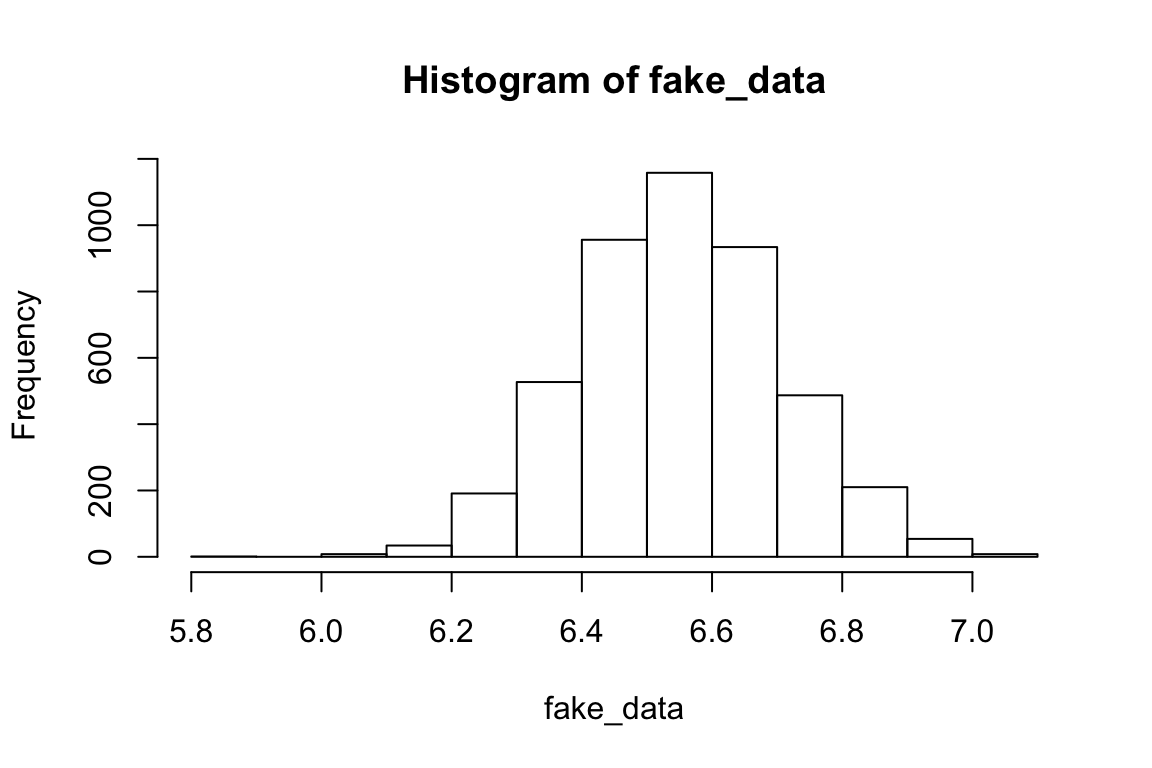A QQ-plot plots the quantiles of 2 distributions against one another and allows direct comparison. If they are the same distribution, the points will lay on top of the line x=y. By default qqplot() takes the argument and compares it to the standard normal. qqnorm(rts$RTlexdec)
qqline(rts$RTlexdec)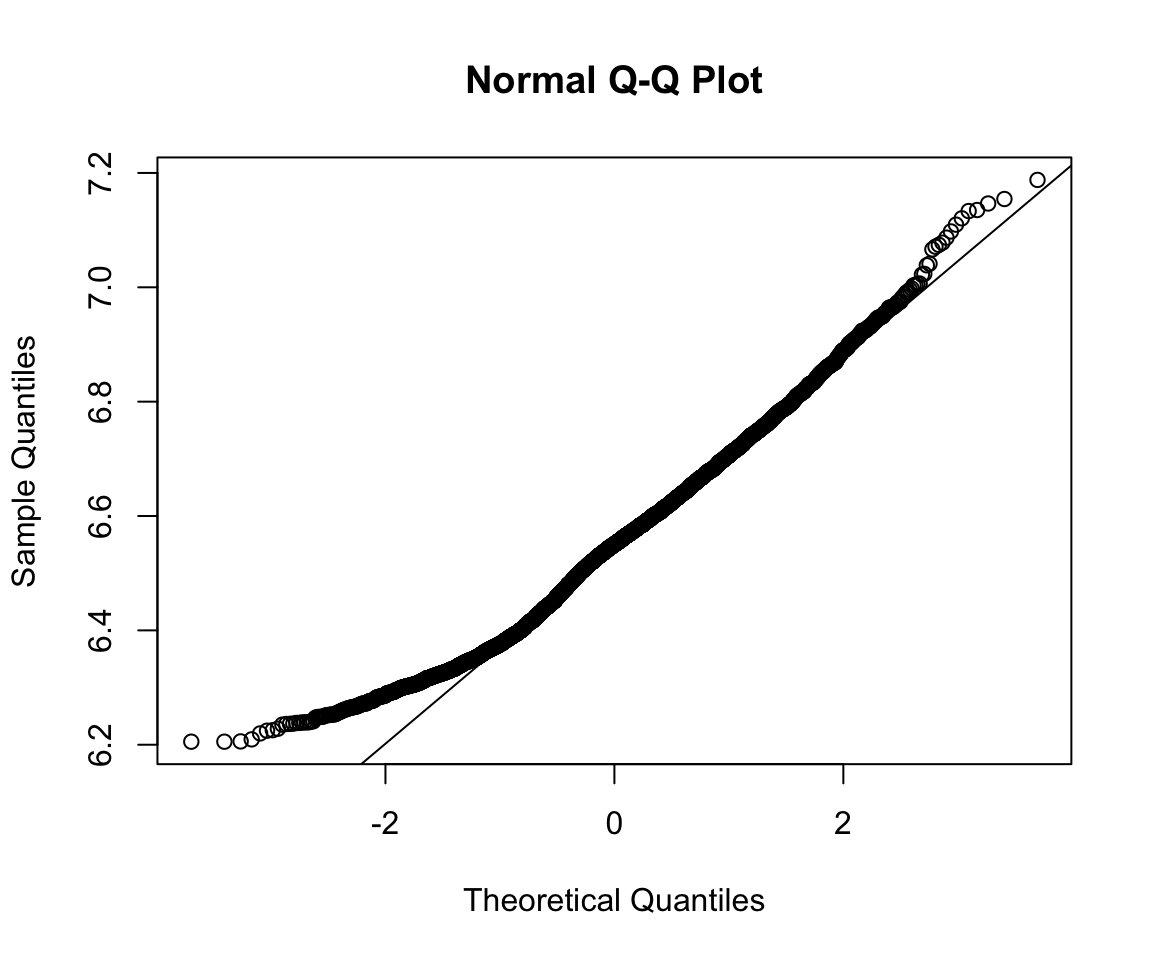qqnorm(fake_data) qqline(fake_data)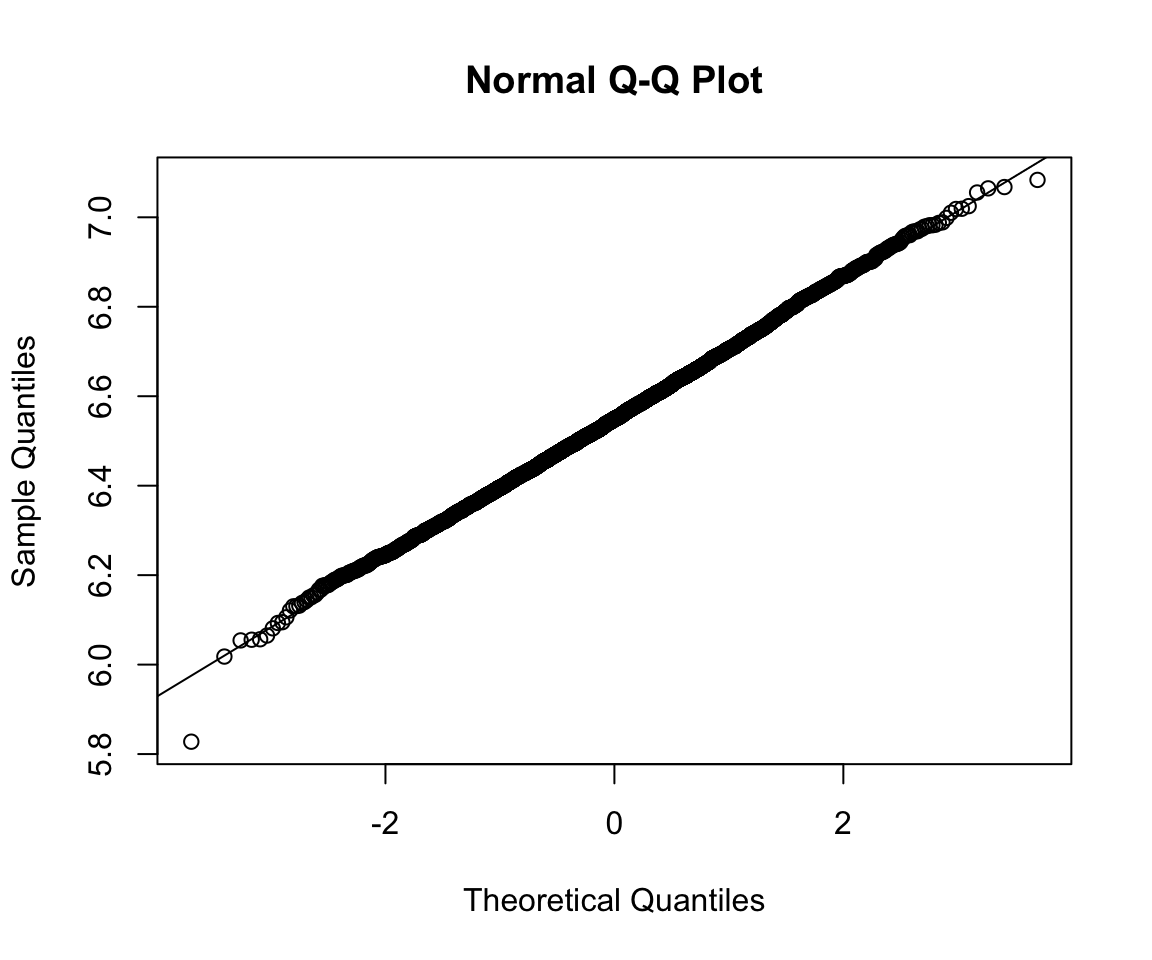### Correlation Going back to our scatterplots of RTs and word lengths. There was some evidence that there was a relationship there that we could see but what if we want to quantify that? The easiest tool for that is the correlation coefficient. • The correlation coefficient measures the strength of linear association between two variables. • It is always between -1 and 1, inclusive, where • -1 indicates perfect negative relationship • 0 indicates no relationship • +1 indicates perfect positive relationship Pearson’s correlation coefficient: $$r_{xy} =\frac{1}{n-1} \sum_{i=1}^{n}(\frac{x_i-\overline{x}}{s_x})\times(\frac{y_i-\overline{y}}{{s_y}})$$ Note that what’s being multiplied is the z-scored x and y rt_data <- rt_data %>% mutate(word_length = str_length(word)) pearson_r1 <- sum(scale(rt_data$word_length) * scale(rt_data$rt)) / (nrow(rt_data) - 1) pearson_r2 <- cor(rt_data$word_length, rt_data\$rt)

Okay, but what does it mean to multiply all the values together like that? Here is an example of uncorrelated variables.

x <- rnorm(1000)
y <- rnorm(1000)
xy <- data_frame(x, y)
ggplot(xy, aes(x, y)) +
geom_point(shape = 1)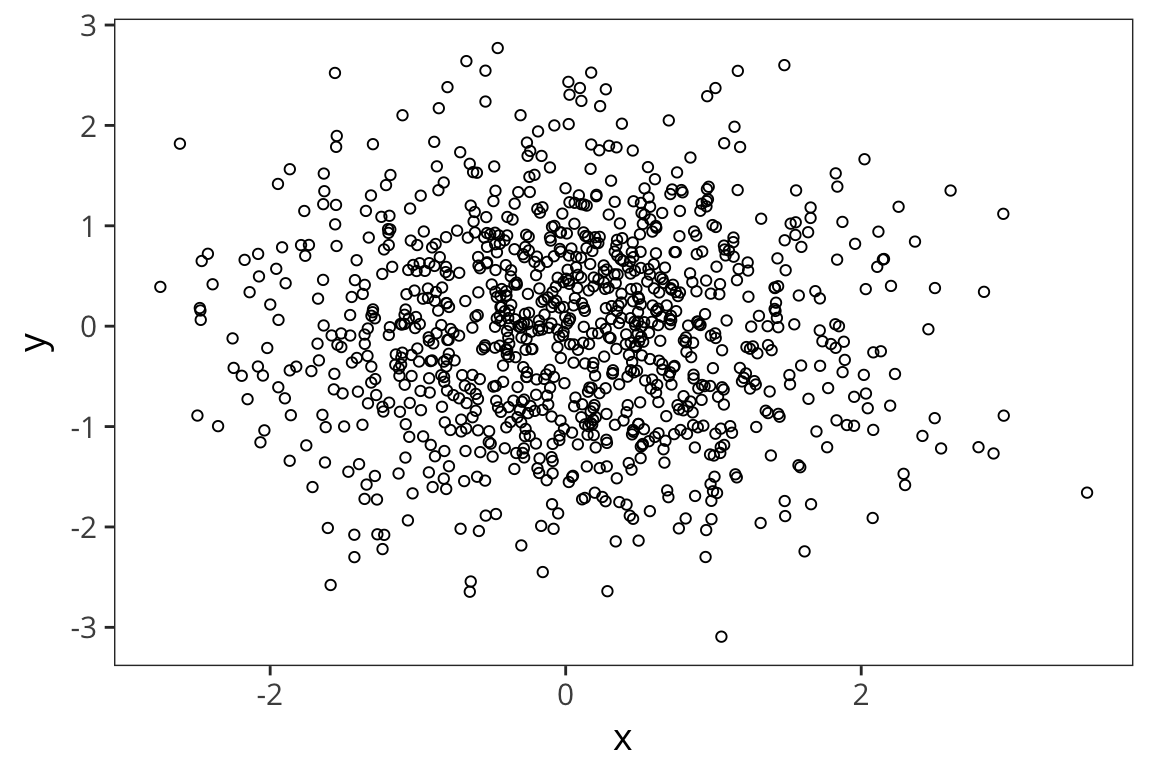sum(scale(x) * scale(y)) / (length(x) - 1)
##  -0.02502228
cor(x, y)
##  -0.02502228

On the other hand:

z <- x
xz <- data_frame(x, z)
ggplot(xz, aes(x, z)) + geom_point(shape = 1)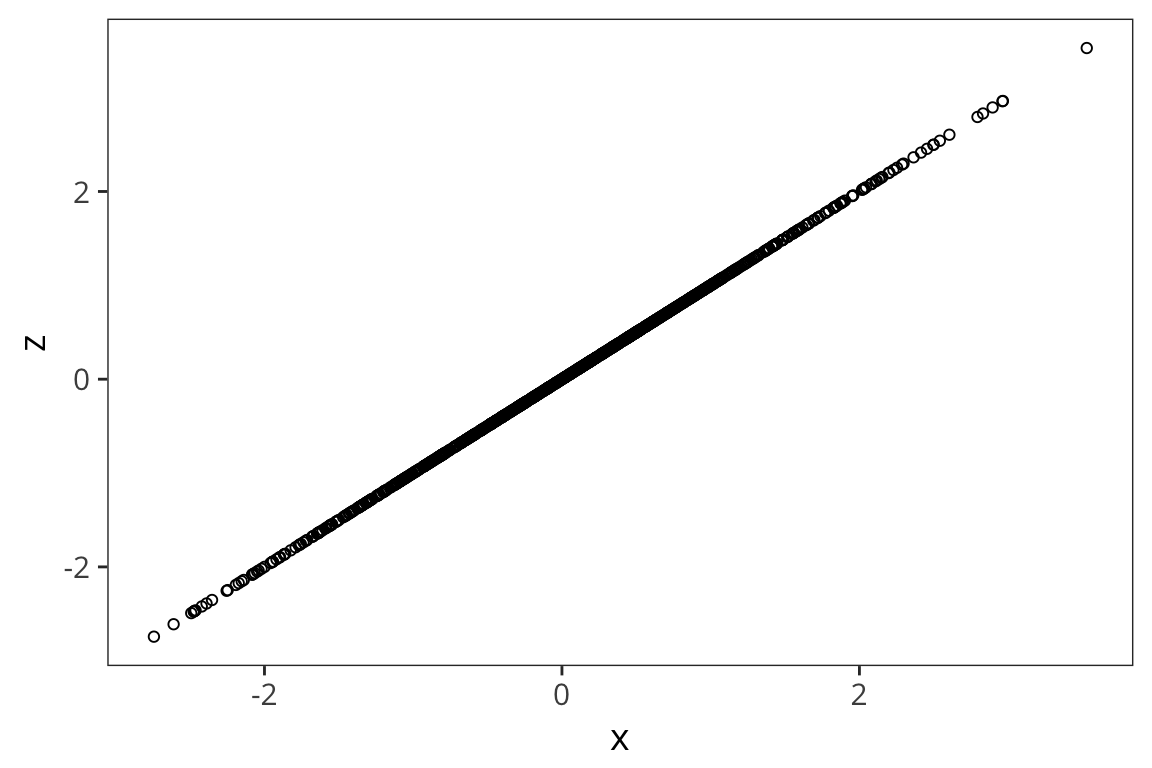sum(scale(x) * scale(z)) / (length(x) - 1)
##  1

To get a better sense of what different correlation coefficients represent, check out the awesome visualization by Kristoffer Magnuson: http://rpsychologist.com/d3/correlation/.

• try different correlations and see the effect of moving outliers
• try different sample sizes and see the effect of outliers